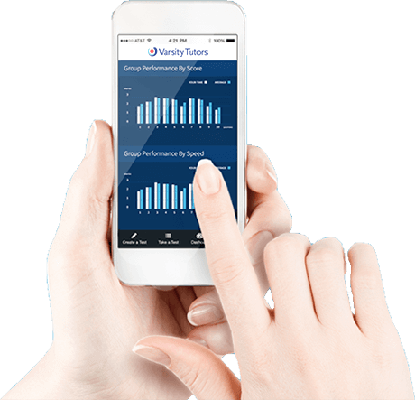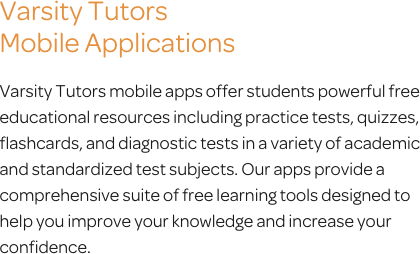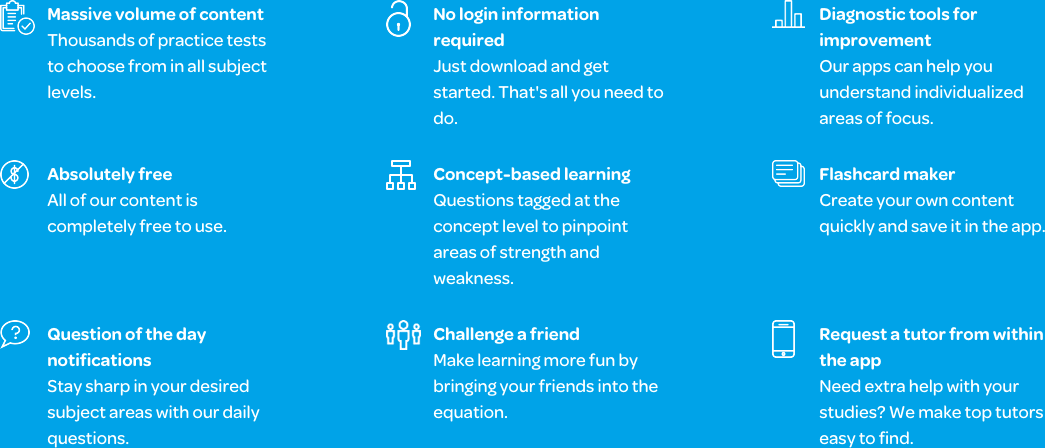# Varsity Tutors Common Core Math 4th Grade mobile app### The Varsity Tutors Common Core Math 4th Grade Mobile App

If you have a child in the 4th grade who is struggling with mathematics or is about to take the Common Core 4th Grade Mathematics exam, the Varsity Tutors Common Core 4th Grade Math mobile learning app might be useful for you. Since the content covered in Common Core curriculum varies from state to state, the material covered in this program will include the most important aspects of the subject, thus ensuring your child gets the proper preparation needed to succeed. Whether it’s multiplication, understanding number values up to 1,000,000, complex geometry, or being able to add and subtract fractions, this program can help them prepare for any upcoming tests.

The Common Core 4th Grade Math app is free and available on iTunes or the Google Play Store. Since the app is designed for mobile devices, you can conveniently turn any moment into a perfect study session. This means that your child is always a button-click away from getting the review they need to ensure they succeed in the subject.

The mobile app contains a number of resources for your child. Thus, it will provide them a balanced study session that can better equip them for any challenges they may face in the classroom. If you want them to keep 4th-grade math in the forefront of their minds, have them solve the Question of the Day every day. This feature generates a different problem every day and can also be used as a way to begin a study session.

For more detailed study sessions, try working with the Learn by Concept feature. Think of this as an interactive textbook. Through the interactive syllabus, the course is divided into topics, which can be accessed by the user. This is a great way to learn about material that hasn’t been taught yet or simply review previously-learned concepts. In addition, your child can also test their knowledge through the many flashcards. Since they are also arranged by topics, you can choose specific sections that are problematic and review them.

There are also a number of practice tests that are available on the mobile learning app. If you want to give your child a comprehensive evaluation, try one of the Full-Length Practice Tests. They are quite long and cover all of the material presented in the course. These tests are designed to simulate the actual test-taking experience, so they are an ideal way to help your student mentally prepare for an upcoming exam. On the other hand, if you are interested in reviewing specific portions of the subject, or you want a quicker evaluation, try the shorter concept-specific practice tests. Here, you can focus on specific areas of your child’s study program and determine which parts of the subject they need to focus more time studying. The practice tests also provide a useful analysis at the end, which tells you the time it took your child to answer the questions and complete the test, a thorough explanation of the correct answers, and a comparison of how your student did in relation to others who took the practice test.

The Varsity Tutors Common Core 4th Grade Math app is available on the iPad, iPhone, and all Android devices. It is portable and easy to use, making it one of the best ways to supplement classroom-based learning. By incorporating this into your child’s study plan, you are working to give them a balanced education that can get them the insight needed to succeed in their class.There are three critical areas that each child’s attention is focused on. They will work with multi-digit multiplication and division, as well as learn to solve problems that have fractions, and work with geometric shapes. It is imperative that each student forms a thorough understanding of the relationships between numbers in order for them to succeed in future math courses. Throughout the fourth grade, they will be tested regularly for their proficiency until they take a cumulative exam at the end of the year.

The Common Core 4th grade math exam uses multiple-choice, short response, and extended response questions to assess a child’s mathematical understanding. Students are required to solve questions that address each concept they face. Multiple choice questions assess their understanding of multi-step problems. The short response questions assess each child’s ability to reason while demonstrating their understanding of concepts. There are often multi-step problems that call for real-world applications while encouraging students to conceptualize the questions. Furthermore, the extended response questions test for conceptual understanding of mathematical applications and strategies. Students may be required to criticize arguments using mathematical reasoning.

At the beginning of the school year, students in the fourth grade are expected to first become proficient in algebraic operations and reasoning. They will need to be able to use all four operations when solving various types of problems. Each child is tasked with solving complex multiplication equations using the properties they learned in the previous year. They will need to be able to use multiplicative comparisons with imagery to solve division and multiplication word and equation problems. In addition, students will learn to use mental math strategies to estimate, reason, and solve problems fluidly. They will have to work with the factors and multiples of numbers one through 100. Students must be familiar with prime and composite numbers. Further, they will learn to create and analyze patterns that involve numbers and shapes.

The following concept fourth graders learn in Common Core 4th grade math is base ten operations and numbers. This means they will work with math problems that require they demonstrate an understanding of manipulating numbers in tens. When working with whole numbers that have multiple digits, students will be required to read and write them in expanded, number, and numeral formats. They will need to be able to compare them using symbols, round numbers to any place, and solve problems using division and place value concepts. Fourth-grade students will need to be able to demonstrate an understanding of the properties of operations and place value. They will use standard math equations to solve multi-digit problems. In addition, they will need to solve multi-digit multiplication problems using whole numbers. There will be some that have as many as four digits, which students will need to solve using their knowledge of strategies and relationships. Furthermore, they will need to be able to demonstrate the reasoning for each answer using explanations and illustrations.

From whole numbers, students can progress to fractions. Though fractions can be difficult for some students to grasp, it is an important aspect of the coursework, and it is just one topic covered in-depth by the Varsity Tutors Common Core Grade 4 Math app for iPhone, iPad, and Android. It is important that each student has a firm understanding of fractional equations and relationships. They must be able to extend their understanding of whole numbers and their relationships to that of fractions. They will use this to create equivalent fractions. In addition, fourth-graders need to be able to make comparisons between fractions that have different numerators and denominators using symbols. During the factoring unit, students will learn to use unit fractions to build fractions. They need to develop an understanding of the strategies involved while adding, subtracting, dividing, or multiplying fractions. They must be able to decompose fractions into different fractions, as well as be able to express them as a sum of fractions. They will need to be able to solve math problems that use mixed numbers, as well as word problems, while using visual models.

From there, the pace changes to focus on decimal notations. Students will need to understand and compare decimals to fractions and notations. They will be expected to express fractions over 10 using the appropriate techniques. They will need to use decimal notation with fractions that have a denominator of 100, as well as make comparisons between decimals to the hundredths place.

Measurement follows fractions, allowing students to progress in their understanding of measurements and their respective conversions. They will be required to develop a grasp of the various measurements and their relative sizes. They will learn to record measurements using the appropriate units, as well as generate conversions based on their list. Students solve distance, time, volume, financial, and mass word problems while demonstrating their responses in illustrations. Furthermore, they will learn to represent the data as they find it, regardless if they are using whole or fractional numbers. They will begin working with angles to identify measurements, shapes, and end points. Students must be able to recognize additive angle measurements.

Finally, students work with geometry to set the basis for future coursework. They will learn to draw and identify two-dimensional figures, such as parallel lines, angles, line segments, and more. These figures will be classified based on certain features that students must be able to identify. By downloading the free Varsity Tutors Common Core Grade 4 Math app from iTunes or the Google Play Store, everything in the curriculum is right at your fingertips.

Though there is a great deal of content to cover in the Common Core 4th Grade Math coursework, students who do well can begin a healthy learning relationship with their future math classes. Each concept builds on the next, allowing students to develop the skills most important to the course. Help your child build their math foundations with the free Common Core Grade 4 Math app from Varsity Tutors. You can download it today for any iPhone, iPad, or Android-powered device.